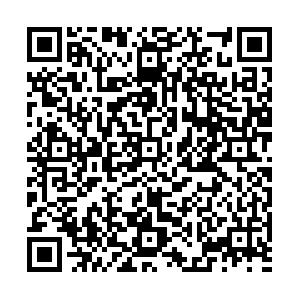# Study of C parity violating and strangeness changing J/ψ → PP weak decays• Although $J/{\psi}$weak decays are rare, they are possible within the standard model of elementary particles. Inspired by the potential prospects of the future intensity frontier, the C parity violating $J/{\psi}$${\to}$${\pi}{\eta}^{({\prime})}$, ${\eta}{\eta}^{\prime}$decays and the strangeness changing $J/{\psi}$${\to}$${\pi}K$, $K{\eta}^{({\prime})}$decays are studied via the perturbative QCD approach. It is determined that the $J/{\psi}$${\to}$${\eta}{\eta}^{\prime}$decays have relatively large branching ratios, approximately on the order of $10^{-11}$, which might be within the measurement capability and sensitivity of the future STCF experiment.
••Get Citation
Yueling Yang, Junliang Lu, Mingfei Duan, Jinshu Huang and Junfeng Sun. Study of the C parity violating and strangeness changing J/ψPP weak decays[J]. Chinese Physics C. doi: 10.1088/1674-1137/ac06ac
Yueling Yang, Junliang Lu, Mingfei Duan, Jinshu Huang and Junfeng Sun. Study of the C parity violating and strangeness changing J/ψPP weak decays[J]. Chinese Physics C.Milestone
Article Metric

Article Views(222)
Cited by(0)
Policy on re-use
To reuse of Open Access content published by CPC, for content published under the terms of the Creative Commons Attribution 3.0 license (“CC CY”), the users don’t need to request permission to copy, distribute and display the final published version of the article and to create derivative works, subject to appropriate attribution.
###### 通讯作者: 陈斌, bchen63@163.com
• 1.

沈阳化工大学材料科学与工程学院 沈阳 110142

Title:
Email:

## Study of C parity violating and strangeness changing J/ψ → PP weak decays

• 1. Institute of Particle and Nuclear Physics, Henan Normal University, Xinxiang 453007, China
• 2. School of Physics and Electronic Engineering, Nanyang Normal University, Nanyang 473061, China

Abstract: Although $J/{\psi}$weak decays are rare, they are possible within the standard model of elementary particles. Inspired by the potential prospects of the future intensity frontier, the C parity violating $J/{\psi}$${\to}$${\pi}{\eta}^{({\prime})}$, ${\eta}{\eta}^{\prime}$decays and the strangeness changing $J/{\psi}$${\to}$${\pi}K$, $K{\eta}^{({\prime})}$decays are studied via the perturbative QCD approach. It is determined that the $J/{\psi}$${\to}$${\eta}{\eta}^{\prime}$decays have relatively large branching ratios, approximately on the order of $10^{-11}$, which might be within the measurement capability and sensitivity of the future STCF experiment.

### HTML关注分享DownLoad:  Full-Size Img  PowerPoint